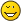EfficiencyEgonDeveloper Posts: 889 Threads: 52 Joined: 2012-06-26 #7 2015-06-14, 21:35 (This post was last modified: 2015-06-14, 22:06 by Egon.) Hi Folks, I have changed the computation of the effectivity shown in the station list at blitzortung.org (this is not the efficiency of lightningmaps.org you have discussed above). The time period is 1 hour. I need the following notations to explain the computation. Strikes (x,s) = number of strikes in a rage of x km to station s Hits (x,s) =  number of strikes in a range of x km to station s at that station s was involved Signals (s) = number of signals of station s Strikes = total number of computed strikes of the region to that the station sends data min (x,y) = minimum of x and y Then we have: Effectivity_50 = Hits (s,50) / Strikes (s,50) * min (Strikes / Signals (s), Signals (s) / Strikes) Effectivity_500 = Hits (s,500) / Strikes (s,500) * min (Strikes / Signals (s) /, Signals (s) / Strikes) Effectivity_5000 = Hits  (s,5000) / Strikes(s,5000) * min (Strikes / Signals (s), Signals (s) / Strikes) If a denominator is zero, the effectivity is zero. This is the old effectivity value multiplied by the factor min (Strikes / Signals(s), Signals(s) / Strikes) to consider also the strikes/signals relation (and to punish the stations that send a lot of trash). The effectivity should only be considered as a relative measure of the performance, although a value of 1 can be obtained. Much fun, Egon Stations: 2656 « Next Oldest | Next Newest »